# RD Sharma Solutions for Class 6 Maths Chapter 20: Mensuration Exercise 20.4

Students would have learnt about the concept of determining area of various structures in the previous exercise. Here, the students mainly gain knowledge about the important formulas and steps which are followed to determine the area of rectangle and square. Each exercise contains a set of illustrative examples to make them familiar with the type of questions that would arise in the exam. Students can refer to the solutions PDF to understand the method of solving problems and improve their speed. Here, the RD Sharma Solutions Class 6 Maths Chapter 20 Mensuration Exercise 20.4 PDF can be accessed by the students from the links, which are provided below.

## RD Sharma Solutions for Class 6 Maths Chapter 20: Mensuration Exercise 20.4 Download PDF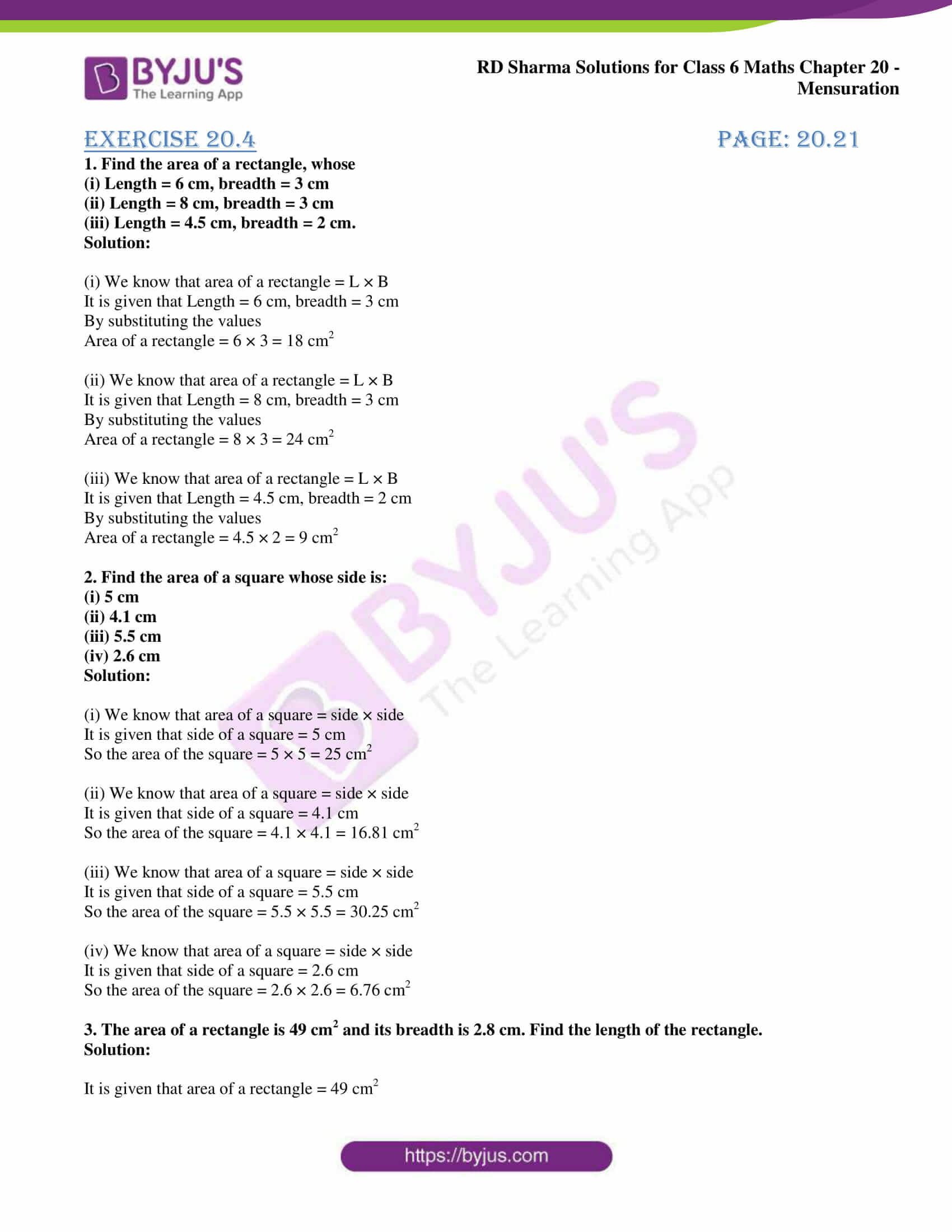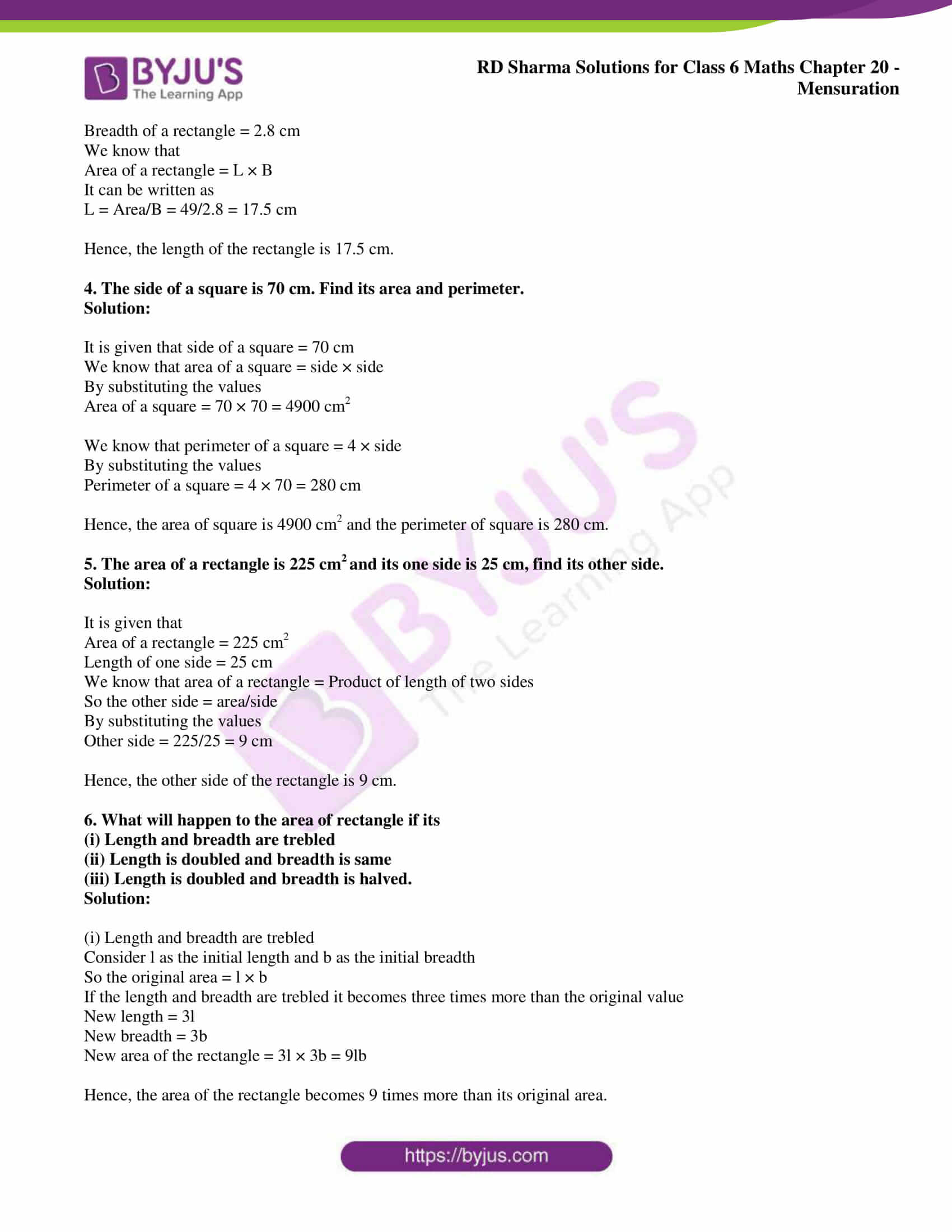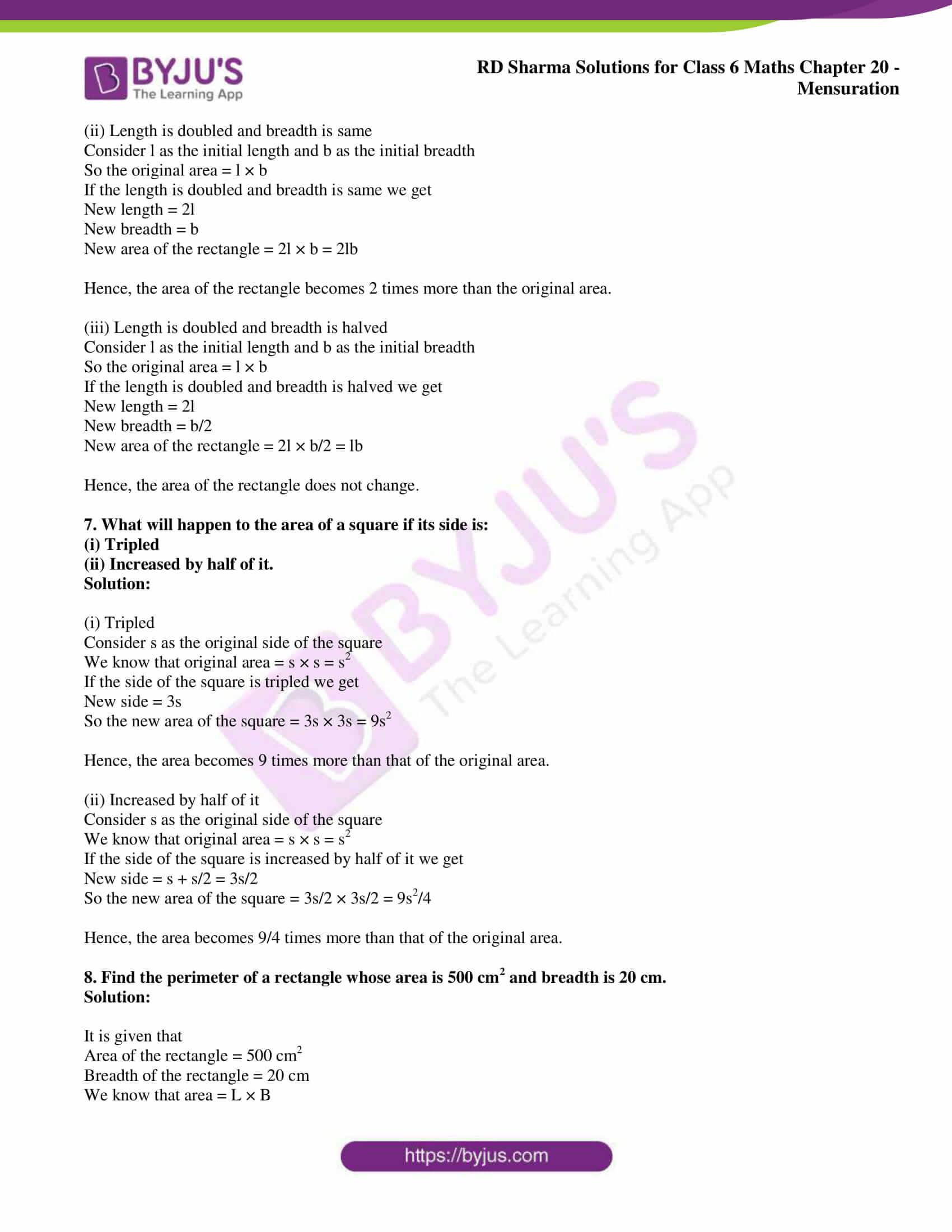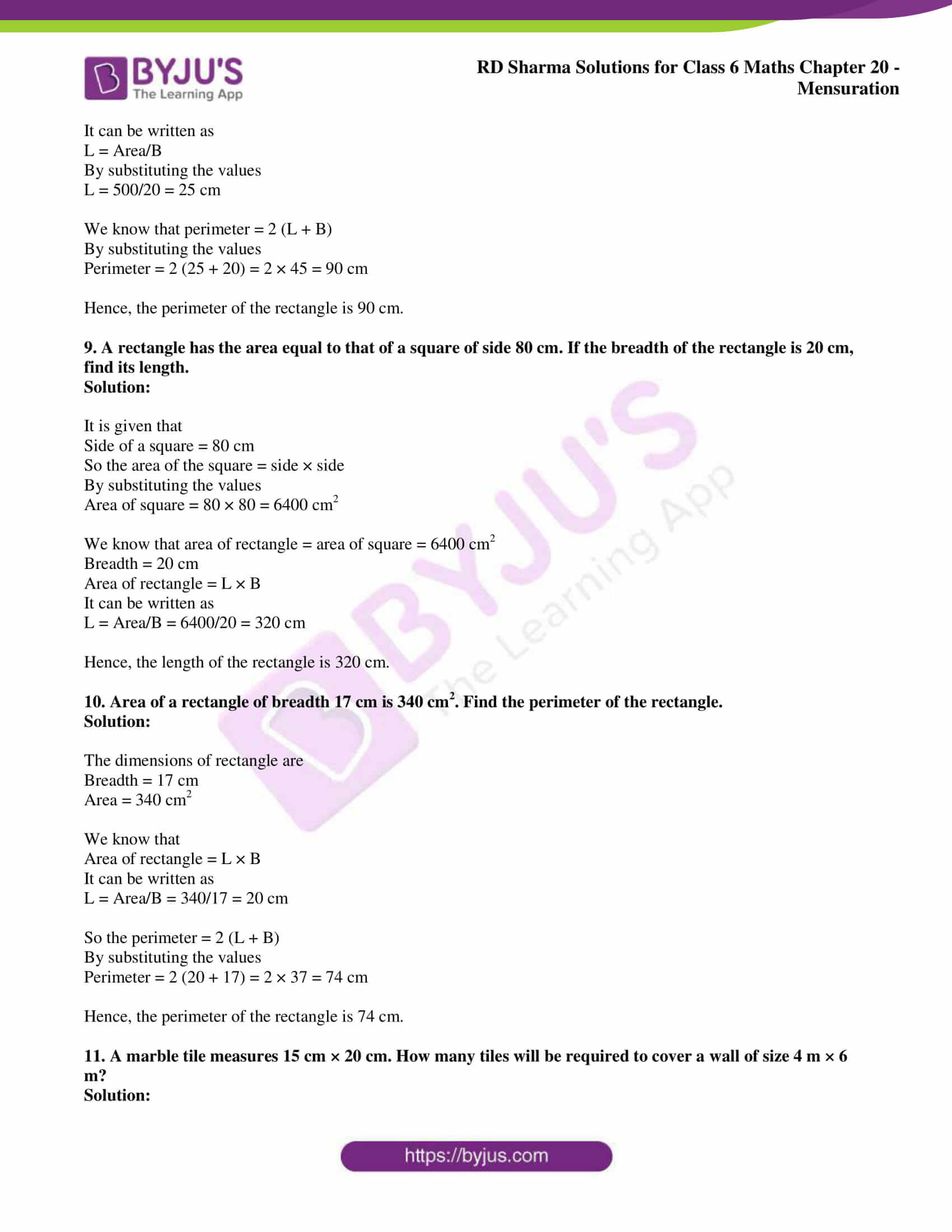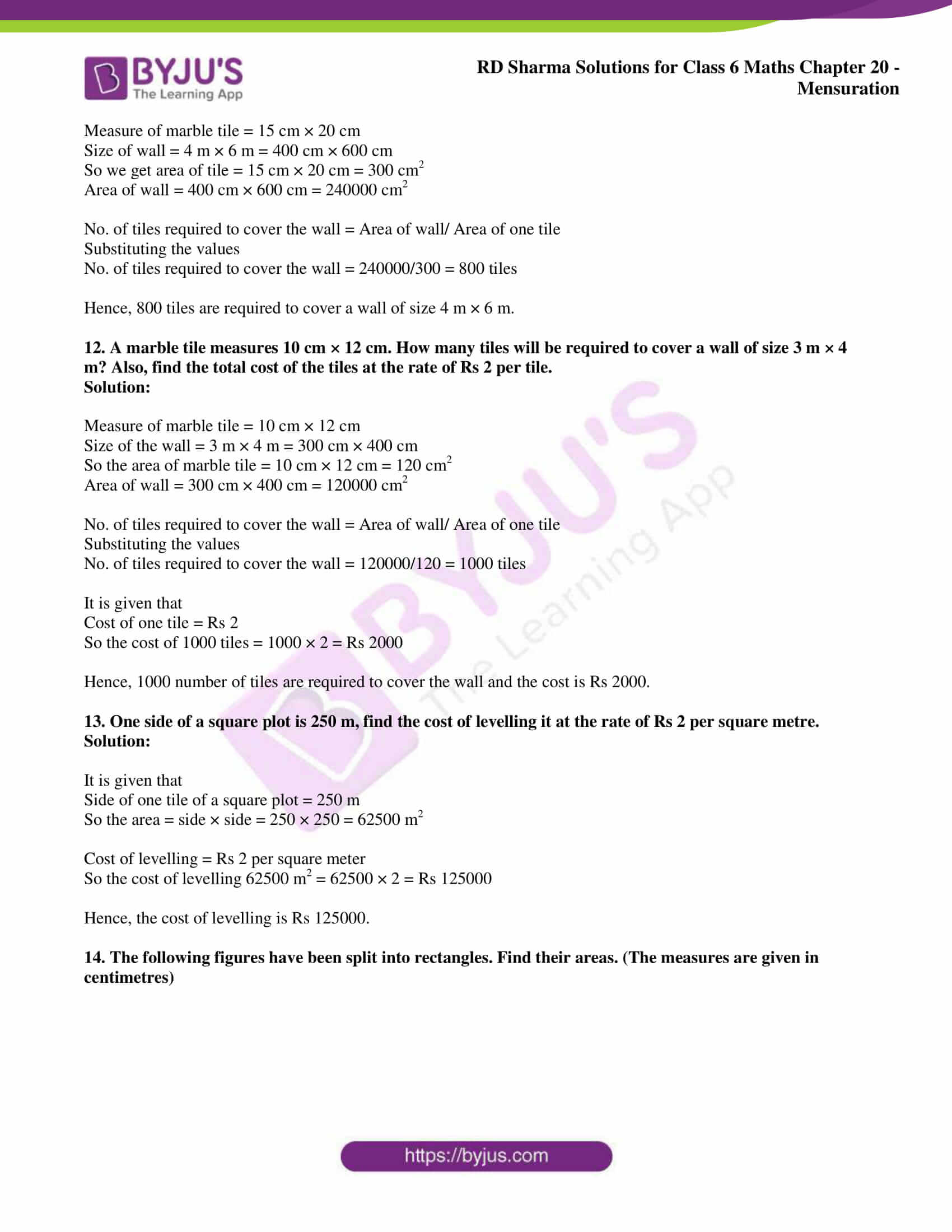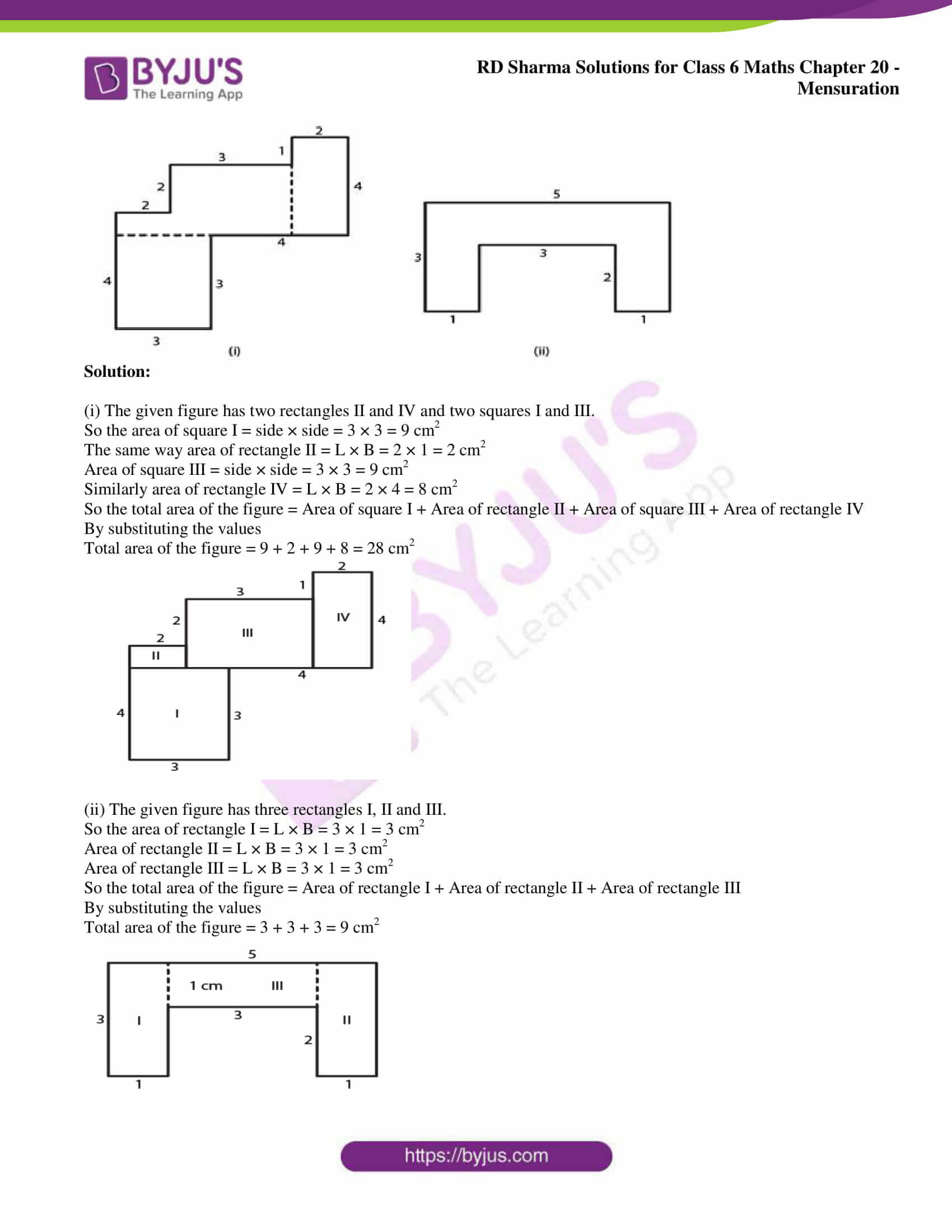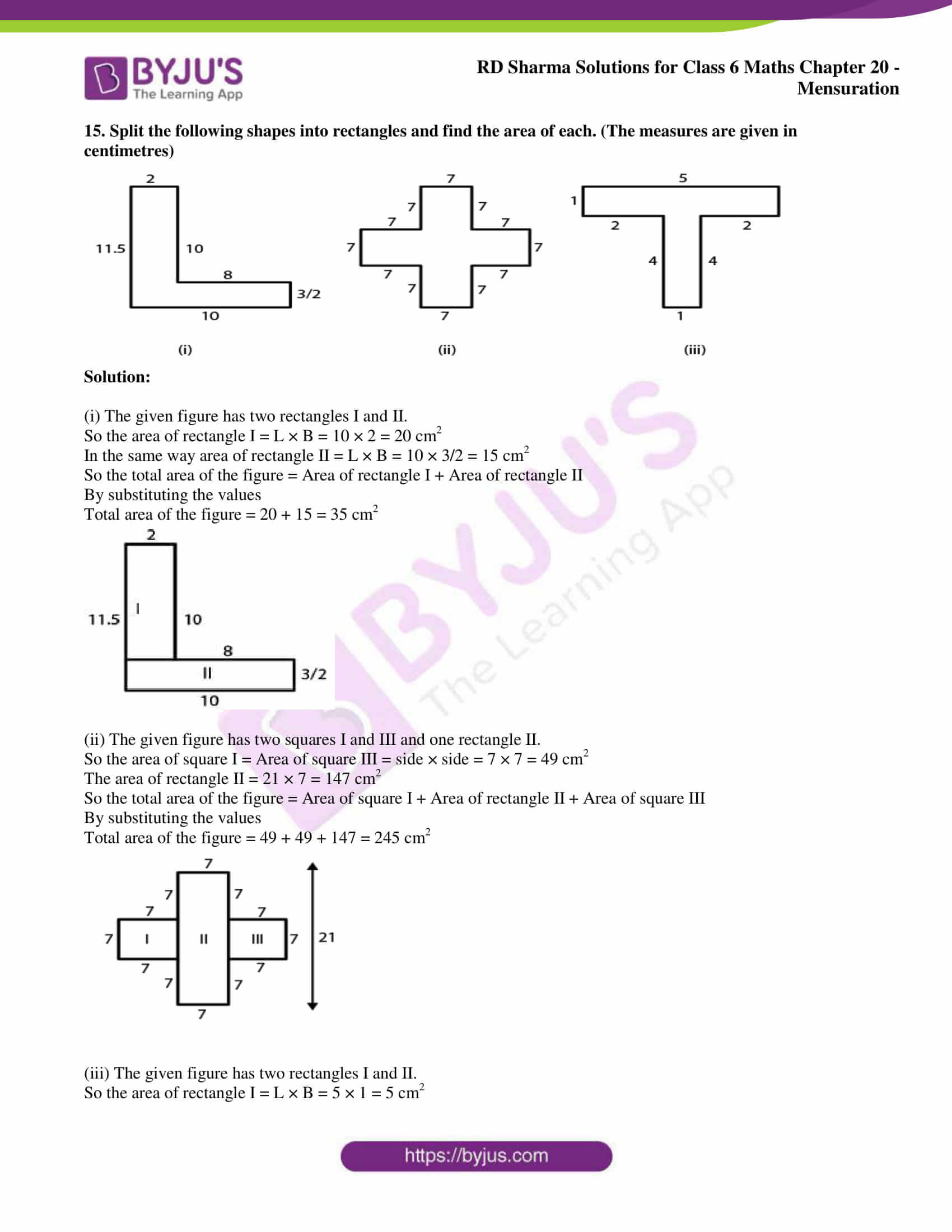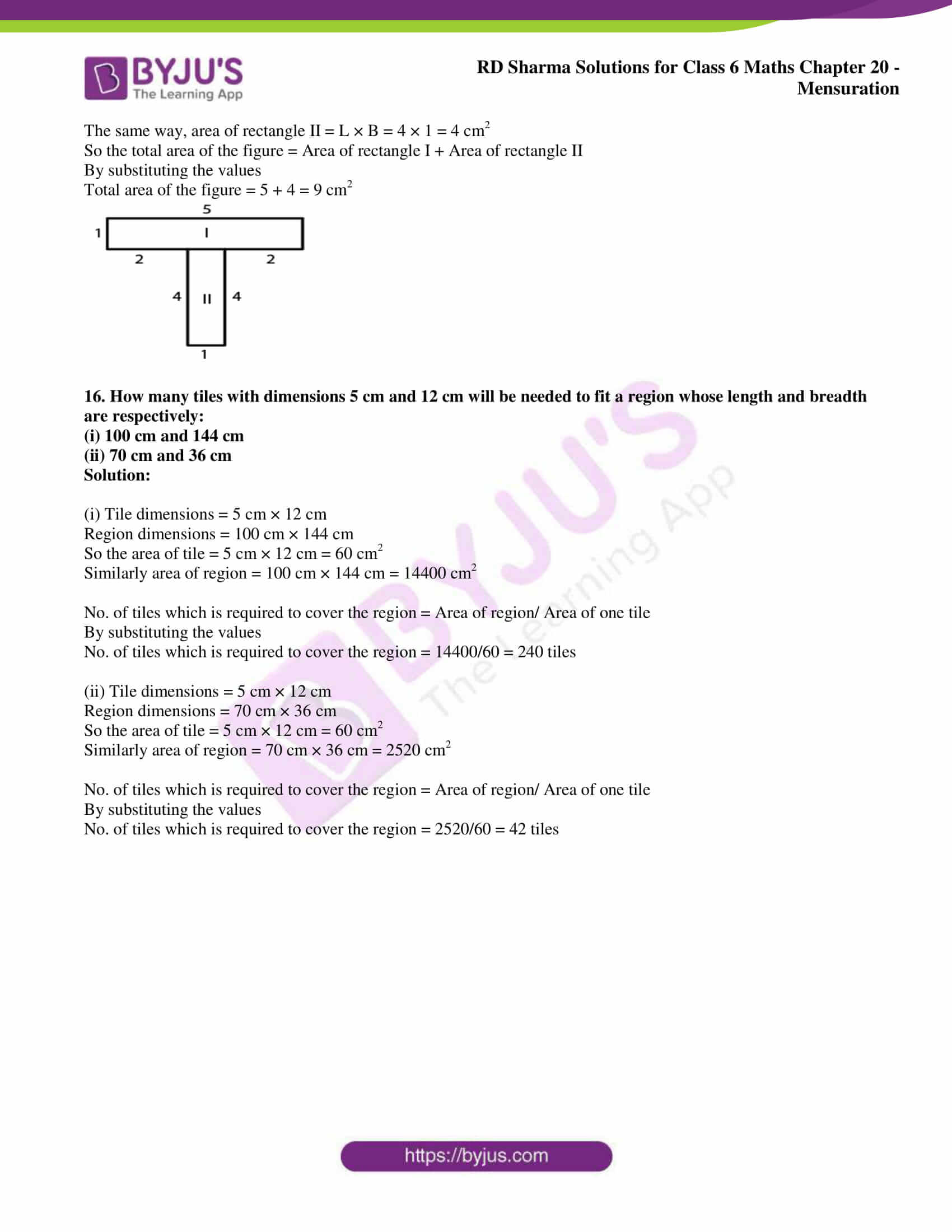### Exercise 20.4 page: 20.21

1. Find the area of a rectangle, whose

(i) Length = 6 cm, breadth = 3 cm

(ii) Length = 8 cm, breadth = 3 cm

(iii) Length = 4.5 cm, breadth = 2 cm.

Solution:

(i) We know that area of a rectangle = L × B

It is given that Length = 6 cm, breadth = 3 cm

By substituting the values

Area of a rectangle = 6 × 3 = 18 cm2

(ii) We know that area of a rectangle = L × B

It is given that Length = 8 cm, breadth = 3 cm

By substituting the values

Area of a rectangle = 8 × 3 = 24 cm2

(iii) We know that area of a rectangle = L × B

It is given that Length = 4.5 cm, breadth = 2 cm

By substituting the values

Area of a rectangle = 4.5 × 2 = 9 cm2

2. Find the area of a square whose side is:

(i) 5 cm

(ii) 4.1 cm

(iii) 5.5 cm

(iv) 2.6 cm

Solution:

(i) We know that area of a square = side × side

It is given that side of a square = 5 cm

So the area of the square = 5 × 5 = 25 cm2

(ii) We know that area of a square = side × side

It is given that side of a square = 4.1 cm

So the area of the square = 4.1 × 4.1 = 16.81 cm2

(iii) We know that area of a square = side × side

It is given that side of a square = 5.5 cm

So the area of the square = 5.5 × 5.5 = 30.25 cm2

(iv) We know that area of a square = side × side

It is given that side of a square = 2.6 cm

So the area of the square = 2.6 × 2.6 = 6.76 cm2

3. The area of a rectangle is 49 cm2 and its breadth is 2.8 cm. Find the length of the rectangle.

Solution:

It is given that area of a rectangle = 49 cm2

Breadth of a rectangle = 2.8 cm

We know that

Area of a rectangle = L × B

It can be written as

L = Area/B = 49/2.8 = 17.5 cm

Hence, the length of the rectangle is 17.5 cm.

4. The side of a square is 70 cm. Find its area and perimeter.

Solution:

It is given that side of a square = 70 cm

We know that area of a square = side × side

By substituting the values

Area of a square = 70 × 70 = 4900 cm2

We know that perimeter of a square = 4 × side

By substituting the values

Perimeter of a square = 4 × 70 = 280 cm

Hence, the area of square is 4900 cm2 and the perimeter of square is 280 cm.

5. The area of a rectangle is 225 cm2 and its one side is 25 cm, find its other side.

Solution:

It is given that

Area of a rectangle = 225 cm2

Length of one side = 25 cm

We know that area of a rectangle = Product of length of two sides

So the other side = area/side

By substituting the values

Other side = 225/25 = 9 cm

Hence, the other side of the rectangle is 9 cm.

6. What will happen to the area of rectangle if its

(i) Length and breadth are trebled

(ii) Length is doubled and breadth is same

(iii) Length is doubled and breadth is halved.

Solution:

(i) Length and breadth are trebled

Consider l as the initial length and b as the initial breadth

So the original area = l × b

If the length and breadth are trebled it becomes three times more than the original value

New length = 3l

New area of the rectangle = 3l × 3b = 9lb

Hence, the area of the rectangle becomes 9 times more than its original area.

(ii) Length is doubled and breadth is same

Consider l as the initial length and b as the initial breadth

So the original area = l × b

If the length is doubled and breadth is same we get

New length = 2l

New area of the rectangle = 2l × b = 2lb

Hence, the area of the rectangle becomes 2 times more than the original area.

(iii) Length is doubled and breadth is halved

Consider l as the initial length and b as the initial breadth

So the original area = l × b

If the length is doubled and breadth is halved we get

New length = 2l

New area of the rectangle = 2l × b/2 = lb

Hence, the area of the rectangle does not change.

7. What will happen to the area of a square if its side is:

(i) Tripled

(ii) Increased by half of it.

Solution:

(i) Tripled

Consider s as the original side of the square

We know that original area = s × s = s2

If the side of the square is tripled we get

New side = 3s

So the new area of the square = 3s × 3s = 9s2

Hence, the area becomes 9 times more than that of the original area.

(ii) Increased by half of it

Consider s as the original side of the square

We know that original area = s × s = s2

If the side of the square is increased by half of it we get

New side = s + s/2 = 3s/2

So the new area of the square = 3s/2 × 3s/2 = 9s2/4

Hence, the area becomes 9/4 times more than that of the original area.

8. Find the perimeter of a rectangle whose area is 500 cm2 and breadth is 20 cm.

Solution:

It is given that

Area of the rectangle = 500 cm2

Breadth of the rectangle = 20 cm

We know that area = L × B

It can be written as

L = Area/B

By substituting the values

L = 500/20 = 25 cm

We know that perimeter = 2 (L + B)

By substituting the values

Perimeter = 2 (25 + 20) = 2 × 45 = 90 cm

Hence, the perimeter of the rectangle is 90 cm.

9. A rectangle has the area equal to that of a square of side 80 cm. If the breadth of the rectangle is 20 cm, find its length.

Solution:

It is given that

Side of a square = 80 cm

So the area of the square = side × side

By substituting the values

Area of square = 80 × 80 = 6400 cm2

We know that area of rectangle = area of square = 6400 cm2

Area of rectangle = L × B

It can be written as

L = Area/B = 6400/20 = 320 cm

Hence, the length of the rectangle is 320 cm.

10. Area of a rectangle of breadth 17 cm is 340 cm2. Find the perimeter of the rectangle.

Solution:

The dimensions of rectangle are

Area = 340 cm2

We know that

Area of rectangle = L × B

It can be written as

L = Area/B = 340/17 = 20 cm

So the perimeter = 2 (L + B)

By substituting the values

Perimeter = 2 (20 + 17) = 2 × 37 = 74 cm

Hence, the perimeter of the rectangle is 74 cm.

11. A marble tile measures 15 cm × 20 cm. How many tiles will be required to cover a wall of size 4 m × 6 m?

Solution:

Measure of marble tile = 15 cm × 20 cm

Size of wall = 4 m × 6 m = 400 cm × 600 cm

So we get area of tile = 15 cm × 20 cm = 300 cm2

Area of wall = 400 cm × 600 cm = 240000 cm2

No. of tiles required to cover the wall = Area of wall/ Area of one tile

Substituting the values

No. of tiles required to cover the wall = 240000/300 = 800 tiles

Hence, 800 tiles are required to cover a wall of size 4 m × 6 m.

12. A marble tile measures 10 cm × 12 cm. How many tiles will be required to cover a wall of size 3 m × 4 m? Also, find the total cost of the tiles at the rate of Rs 2 per tile.

Solution:

Measure of marble tile = 10 cm × 12 cm

Size of the wall = 3 m × 4 m = 300 cm × 400 cm

So the area of marble tile = 10 cm × 12 cm = 120 cm2

Area of wall = 300 cm × 400 cm = 120000 cm2

No. of tiles required to cover the wall = Area of wall/ Area of one tile

Substituting the values

No. of tiles required to cover the wall = 120000/120 = 1000 tiles

It is given that

Cost of one tile = Rs 2

So the cost of 1000 tiles = 1000 × 2 = Rs 2000

Hence, 1000 number of tiles are required to cover the wall and the cost is Rs 2000.

13. One side of a square plot is 250 m, find the cost of levelling it at the rate of Rs 2 per square metre.

Solution:

It is given that

Side of one tile of a square plot = 250 m

So the area = side × side = 250 × 250 = 62500 m2

Cost of levelling = Rs 2 per square meter

So the cost of levelling 62500 m2 = 62500 × 2 = Rs 125000

Hence, the cost of levelling is Rs 125000.

14. The following figures have been split into rectangles. Find their areas. (The measures are given in centimetres)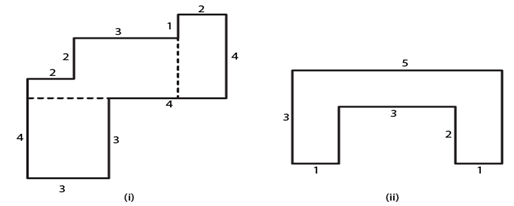Solution:

(i) The given figure has two rectangles II and IV and two squares I and III.

So the area of square I = side × side = 3 × 3 = 9 cm2

The same way area of rectangle II = L × B = 2 × 1 = 2 cm2

Area of square III = side × side = 3 × 3 = 9 cm2

Similarly area of rectangle IV = L × B = 2 × 4 = 8 cm2

So the total area of the figure = Area of square I + Area of rectangle II + Area of square III + Area of rectangle IV

By substituting the values

Total area of the figure = 9 + 2 + 9 + 8 = 28 cm2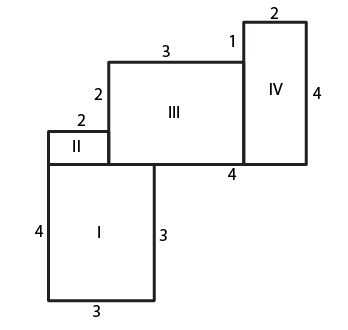(ii) The given figure has three rectangles I, II and III.

So the area of rectangle I = L × B = 3 × 1 = 3 cm2

Area of rectangle II = L × B = 3 × 1 = 3 cm2

Area of rectangle III = L × B = 3 × 1 = 3 cm2

So the total area of the figure = Area of rectangle I + Area of rectangle II + Area of rectangle III

By substituting the values

Total area of the figure = 3 + 3 + 3 = 9 cm2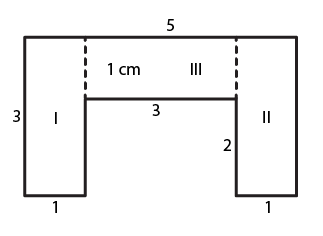15. Split the following shapes into rectangles and find the area of each. (The measures are given in centimetres)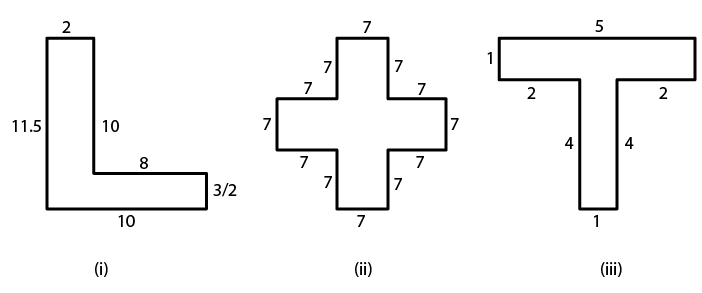Solution:

(i) The given figure has two rectangles I and II.

So the area of rectangle I = L × B = 10 × 2 = 20 cm2

In the same way area of rectangle II = L × B = 10 × 3/2 = 15 cm2

So the total area of the figure = Area of rectangle I + Area of rectangle II

By substituting the values

Total area of the figure = 20 + 15 = 35 cm2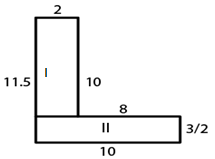(ii) The given figure has two squares I and III and one rectangle II.

So the area of square I = Area of square III = side × side = 7 × 7 = 49 cm2

The area of rectangle II = 21 × 7 = 147 cm2

So the total area of the figure = Area of square I + Area of rectangle II + Area of square III

By substituting the values

Total area of the figure = 49 + 49 + 147 = 245 cm2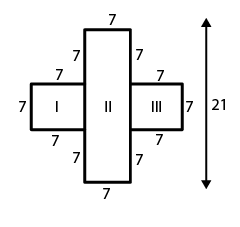(iii) The given figure has two rectangles I and II.

So the area of rectangle I = L × B = 5 × 1 = 5 cm2

The same way, area of rectangle II = L × B = 4 × 1 = 4 cm2

So the total area of the figure = Area of rectangle I + Area of rectangle II

By substituting the values

Total area of the figure = 5 + 4 = 9 cm2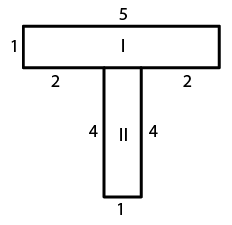16. How many tiles with dimensions 5 cm and 12 cm will be needed to fit a region whose length and breadth are respectively:

(i) 100 cm and 144 cm

(ii) 70 cm and 36 cm

Solution:

(i) Tile dimensions = 5 cm × 12 cm

Region dimensions = 100 cm × 144 cm

So the area of tile = 5 cm × 12 cm = 60 cm2

Similarly area of region = 100 cm × 144 cm = 14400 cm2

No. of tiles which is required to cover the region = Area of region/ Area of one tile

By substituting the values

No. of tiles which is required to cover the region = 14400/60 = 240 tiles

(ii) Tile dimensions = 5 cm × 12 cm

Region dimensions = 70 cm × 36 cm

So the area of tile = 5 cm × 12 cm = 60 cm2

Similarly area of region = 70 cm × 36 cm = 2520 cm2

No. of tiles which is required to cover the region = Area of region/ Area of one tile

By substituting the values

No. of tiles which is required to cover the region = 2520/60 = 42 tiles